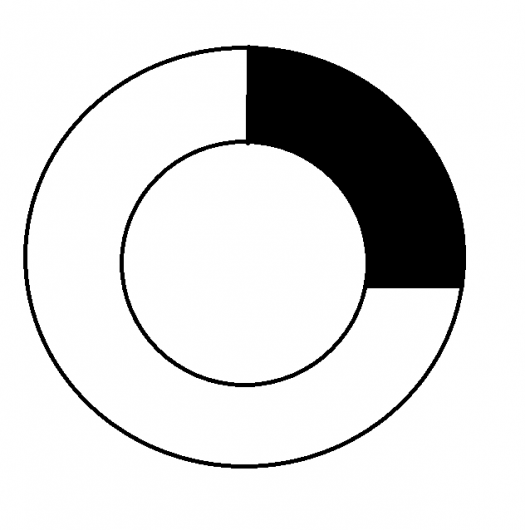# How do I work out the area?### Re: How do I work out the area?

of the shaded area sorry### Re: How do I work out the area?

If R = radius of outer circle and r = radius of inner circle, the shaded area can be fround by $A = \frac{1}{4}\pi (R^2 - r^2)$, assuming R and r are given directly or indirectly.

## Add new comment

### Deafult Input

• Allowed HTML tags: <img> <em> <strong> <cite> <code> <ul> <ol> <li> <dl> <dt> <dd> <sub> <sup> <blockquote> <ins> <del> <div>
• Web page addresses and e-mail addresses turn into links automatically.
• Lines and paragraphs break automatically.
• Mathematics inside the configured delimiters is rendered by MathJax. The default math delimiters are $$...$$ and $...$ for displayed mathematics, and $...$ and $...$ for in-line mathematics.

### Plain text

• No HTML tags allowed.
• Lines and paragraphs break automatically.